Consider Indefinite Admittance Matrix of a linear network with n terminals as shown in the Fig. 5.26.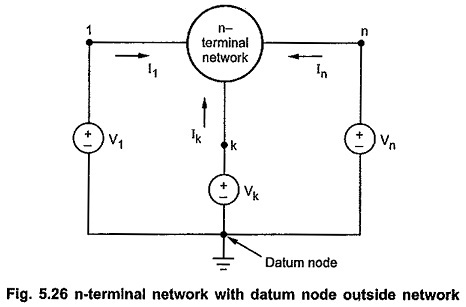Let us consider a zero potential reference node or datum node arbitrarily outside the n-terminal network. Let the voltage sources connected between the n-terminals of the network and datum node be V1,V2,V3, … Vn. Let the currents entering the terminals be I1,I2,I3, … In respectively.

As a network is linear and there are n voltage sources, we can apply superposition theorem for the calculation of current entering each terminal. The current entering each terminals is the partial combination of n sources. The currents are given by,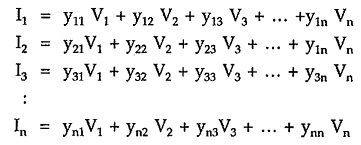Above equations resemble short circuit admittance parameters, there is a basic difference between these parameters and the short circuit admittance parameters of a general two port network. The difference is that the parameters are calculated by considering datum node or reference node outside n-terminal network. Above equations can be written in matrix form as,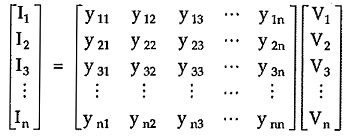In general,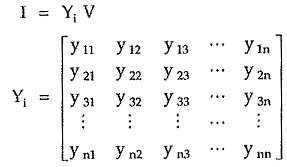The matrix Yi is known as indefinite admittance matrix.

Let yjk be the element of Yi and it is given by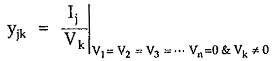The parameter yjk is the ratio of current entering jth terminal when all the node are shorted to reference node except kth node to the voltage at kth node. To determine yjk,

• Short all the terminals to datum node except the terminal k.
• Connect known voltage source Vk between kth terminal and datum node.
• Measure current entering jth terminal as Ij and find ratio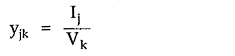### Properties:

The properties of indefinite admittance matrix are as follows,

• The sum of the elements of every column of an indefinite admittance matrix is zero.
• The sum of the elements of every row of an indefinite matrix is zero.
• If an isolated node is added to n-terminal network then the order of indefinite admittance matrix becomes (n + 1) x (n + 1) and it is obtained by adding a row and column of zeros to n x n matrix of original network.
• The value of determinant of indefinite admittance matrix is zero.
• For a reciprocal n-terminal network, indefinite admittance matrix is symmetric.
• If any one the nodes of n-terminal network is made reference node, then the row and the column corresponding to that terminal may be removed from the indefinite admittance matrix.

Scroll to Top